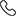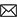Sales :9214233303 | 7240013135 / Tech. Support Desk :7231890000 (9AM ~ 6PM)# CBSE Differential Equations for Class 12th (NCERT) by MC Sir

• Differential Equations are the language in which the laws of nature are expressed. Understanding properties of solutions of differential equations is fundamental to much of contemporary science and engineering. Ordinary differential equations (ODE's) deal with functions of one variable, which can often be thought of as time.
• In this chapter, we will study some basic concepts related to differential equation,general and particular solutions of a differential equation, formation of differential equations, some methods to solve a first order - first degree differential equation and some applications of differential equations in different areas.
• Rs.1,000
• Rs.1,000
• Rs.2,000
Subject Medium Target Language Mathematics English XII Class English + Hindi Topic Based 8 Videos 0 Videos PDF Files Full coverage of topics Differential Equations 12th class(N.C.E.R.T.) from the latest CBSE syllabus All questions fully solved of (Differential Equations)Exemplar Exercise Properly organized solutions in chapter-wise and page wise format help find your solution quickly Step-by-step model solutions to all question Helpful aid during exam preparation Class 11th students who are willing to go an extra mile to study Class 12th concepts in 11th itself. Class 12th students who are keen to pursue a course which covers 12th standard mathematics comprehensively and enables them to fulfill their dream of getting into IITs Essential for CBSE, NIOS & ICSE Exam preparations.

### Other Courses by Manoj Chauhan SirTap a Star to Rate us:
close
close